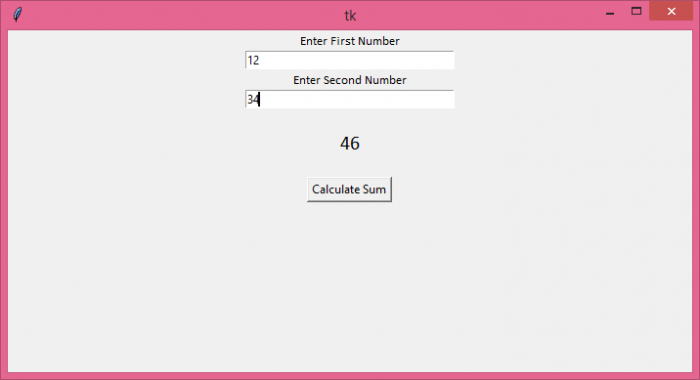# How to change Entry.get() into an integer in Tkinter?

The Entry widget in Tkinter is generally used to accept one-line input in the text field. We can get the output from the Entry widget using .get() method. However, the .get() method returns the output in string format. For example, if the user types an integer number in the Entry widget, it gets converted to a string. To change the type of the Entry input into an integer, we can cast the string to an integer.

## Example

In this example, we have shown how to calculate the sum while taking an integer input from the user.

# Import the required libraries
from tkinter import *

# Create an instance of tkinter frame or window
win=Tk()

# Set the size of the tkinter window
win.geometry("700x350")

def cal_sum():
t1=int(a.get())
t2=int(b.get())
sum=t1+t2
label.config(text=sum)

# Create an Entry widget
Label(win, text="Enter First Number", font=('Calibri 10')).pack()
a=Entry(win, width=35)
a.pack()
Label(win, text="Enter Second Number", font=('Calibri 10')).pack()
b=Entry(win, width=35)
b.pack()

label=Label(win, text="Total Sum : ", font=('Calibri 15'))
win.mainloop()